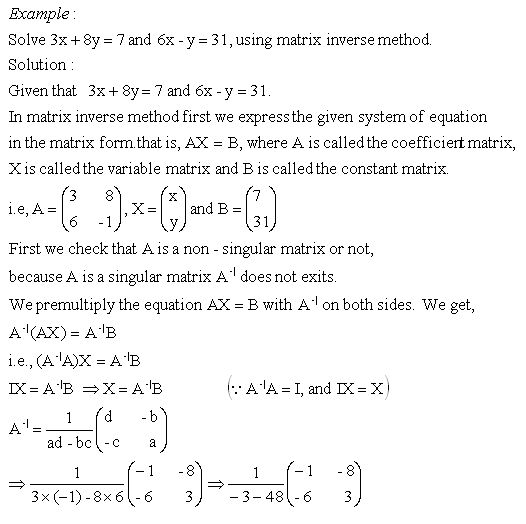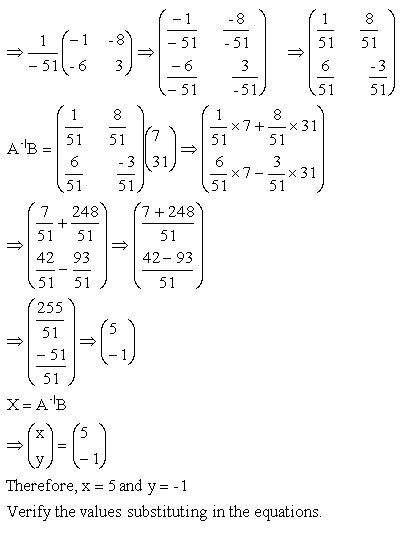Name: ___________________Date:___________________

 Email us to get an instant 20% discount on highly effective K-12 Math & English kwizNET Programs!

### High School Mathematics - 26.6 Solving Linear Equations Using Inverse MethodDirections: Solve the following probelms using above method. Also write at least five examples of your own.
 Q 1: Solve, x + 3y = 11 and 3x + 4y = 18.x = 2 and y = 3x = 2 and y = 4x = 3 and y = 2x = 4 and y = 3 Q 2: Solve, x + 2y = 10 and 3x + 4y = 22.x = 2 and y = 4x = 4 and y = 2x = 2 and y = 3x = 3 and y = 4 Q 3: Solve, x + 3y = 7 and 3x + 4y = 11.x = 1 and y = 3x = 2 and y = 2x = 1 and y = 2x = 2 and y = 1 Q 4: Solve, x + 2y = 8 and 2x + 3y = 13.x = 2 and y = 2x = 2 and y = 5x = 3 and y = 2x = 2 and y = 3 Q 5: Solve, x + 3y = 10 and 3x + 2y = 9.x = 1 and y = 2x = 2 and y = 3x = 1 and y = 3x = 4 and y = 3 Q 6: Solve, 5x + 2y = 24 and x + 3y = 10.x = 3 and y = 1x = 3 and y = 2x = 4 and y = 2x = 4 and y = 3 Question 7: This question is available to subscribers only! Question 8: This question is available to subscribers only!